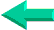### This is an excerpt from Pierre Leclerc (www.excel-vba.com)

The 2 Most Important

Excel Functions AND
Formulas

SUMPRODUCT

AND

INDEX/MATCH

## Lesson 18: Absolute and Relative References in Excel

You need to learn about this concept of absolute and relative references when you start developing large formulas that you want to copy/paste. There is no progress possible in mastering functions and formulas without using the concept of absolute and relative references. The concept is easy to understand and will allow you to develop powerful reporting and analysis applications in Excel.

A Tip: If you already know about absolute and relative reference you may not know about this tip. To add dollar signs to your formulas go to the formula bar, select an address and click on the F4 key at the top of your keyboard. Click once and both the column letter and the row number become absolute, click a second time and the column letter resumes the relative state, click a third time and the row number resumes the relative state, click a forth time and both the column letter and the row number become relative again.

## What is Absolute and Relative References in Excel?

Try this exercise to easily understand the concept. In cell A1 of a new spreadsheet enter 9 and in cell B1 enter the formula =A1. The result is 9 because B1 equal A1.. When there are no dollar signs before the column letter and the row number the address is said to be relative. This means that if you copy/paste cell B1 one cell below in cell B2 the formula becomes =A2 ...the row number in the formula has changed and the result is 0. Now copy/paste cell B1 two cells to the right (in D1) and the formula becomes =C1 the column letter changes and the result becomes 0.

You now know how a relative address acts when it is copy/pasted.

In the next exercise enter 9 in cell A1 and =\$A\$1 in cell B1. The result is 9 because B1 is equal to A1. Now copy/paste cell B1 in cell G6 and notice that the formula remains =\$A\$1 and the results remains 9. The address reference is absolute and the result will not change wherever you copy the formula.

We have seen 2 formulas where the column letter AND the row number are absolute or relative. You can also have formulas where only the column letter is absolute (=\$A1) or where only the row letter is absolute (=A\$1). All four forms can be useful when you start using functions like SUMPRODUCT, INDEX/MATCH, SUMIF, VLOOKUP, ADDRESS, OFFSET and others.

You are now ready to use the most powerful and useful function in Excel SUMPRODUCT

SUMPRODUCT  ¦  INDEX/MATCH  ¦  SUBTOTAL  ¦  ISERROR  ¦  HLOOKUP  ¦  LOOKUP  ¦  VLOOKUP
AVERAGEIF  ¦  AVERAGEIFS ¦  COUNTIF  ¦  COUNTIFS  ¦  SUMIF  ¦  SUMIFS  ¦  IFERRORBack Home